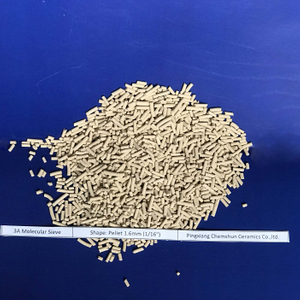+86-7996790781  office@chemshun.com
You are here: » » » 3A Molecular SieveShare to:

# 3A Molecular Sieve

• Chemshun

Production Description:

Molecular sieve 3A is an alkali metal aluminosilicate, it is the potassium form of the type a crystal structure, molecular sieve 3A has an effective pore opening of about 3 angstroms, it absorbs molecule with effective diameters smaller than approximately 3 angstroms, but excludes molecule such as ansaturated hydrocarbons.

Application:

Dehydration of unsaturated Hydrocarbon

Dehydration of cracked petroleum gas in ethylene in industry

Dehydration of natural gas and prevent the formation of COS,

Dehydration of polar liquid

Dehydration of anhydrous alcohol

Static dehydration of insulation glass units

Dehydration of kerosene oil and jet fuel

Material:

Type:3A ; Pore diameter3 angstroms; chemical formula:2/3K2O~1/3Na2O~AL2O3~2SiO2~9/2H2O(SiO2/A2O3=2)

Technical Datas:

 Shape Bead Pellet Diameter(mm) 1.6-2.5(8x12 mesh) 3-5（4x8mesh） 1.6(1/16”) 3.2(1/8”) Static water adsorption(% wt) >=21 >=21 >=21 >=21 Ethylene adsortion(mg/g) <=3 <=3 <=3 <=3 Bulk density(g/ml) >=0.7 >=0.68 >=0.68 >=0.66 Crush strength(N) >=30 >=80 >=35 >=60 Attrition rate(%wt) <=0.2 <=0.2 <=0.25 <=0.25 Size ratio up to grade(%) >=98 >=98 >=96 >=96 Package moisture content(%wt) <=1.5 <=1.5 <=1.5 <=1.5

Production Description:

Molecular sieve 3A is an alkali metal aluminosilicate, it is the potassium form of the type a crystal structure, molecular sieve 3A has an effective pore opening of about 3 angstroms, it absorbs molecule with effective diameters smaller than approximately 3 angstroms, but excludes molecule such as ansaturated hydrocarbons.

Application:

Dehydration of unsaturated Hydrocarbon

Dehydration of cracked petroleum gas in ethylene in industry

Dehydration of natural gas and prevent the formation of COS,

Dehydration of polar liquid

Dehydration of anhydrous alcohol

Static dehydration of insulation glass units

Dehydration of kerosene oil and jet fuel

Material:

Type:3A ; Pore diameter3 angstroms; chemical formula:2/3K2O~1/3Na2O~AL2O3~2SiO2~9/2H2O(SiO2/A2O3=2)

Technical Datas:

 Shape Bead Pellet Diameter(mm) 1.6-2.5(8x12 mesh) 3-5（4x8mesh） 1.6(1/16”) 3.2(1/8”) Static water adsorption(% wt) >=21 >=21 >=21 >=21 Ethylene adsortion(mg/g) <=3 <=3 <=3 <=3 Bulk density(g/ml) >=0.7 >=0.68 >=0.68 >=0.66 Crush strength(N) >=30 >=80 >=35 >=60 Attrition rate(%wt) <=0.2 <=0.2 <=0.25 <=0.25 Size ratio up to grade(%) >=98 >=98 >=96 >=96 Package moisture content(%wt) <=1.5 <=1.5 <=1.5 <=1.5